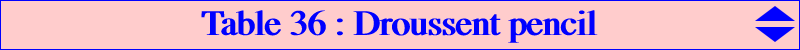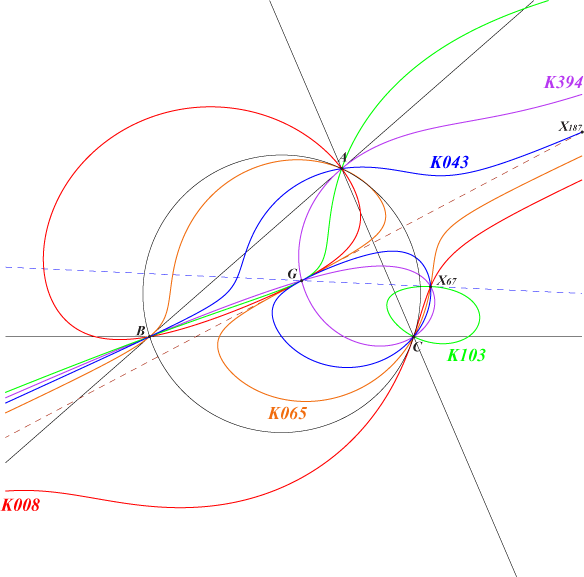The Droussent cubic K008 and the Droussent medial cubic K043 are two circular pivotal cubics which generate a pencil of cubics we call the Droussent pencil. All these cubics contains A, B, C, the circular points at infinity, G, X67, X524 (at infinity) and are tangent at G to the line G, X187 with the exception of K394. Their real asymptotes are parallel to the line GK. Their singular foci lie on a same line through G and the midpoint of O, X111. This pencil contains : – a cubic decomposed into the line at infinity and a circum conic passing through the points G, X67 and X599. – a third pK which is K103 = pK(X67, X67). – a central focal cubic with center G which is K065, the orthopivotal cubic O(X524). – a nodal cubic with node G which is K394. – two other focal cubics which are real when the triangle ABC is acutangle. – two nKs with complicated equations.   See a generalization in CL051.